Do you like the article?
Share it with others -

Use new possibilities of MetaTrader 5

# Studying candlestick analysis techniques (part IV): Updates and additions to Pattern Analyzer

15 May 2019, 16:09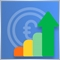5
14 067

### Introduction

In earlier articles within this series we created a MetaTrader 5 app, which tested the relevance of existing candlestick patterns. A later version featured the possibility to create custom patterns based on simple candlestick types, such as short and long candles, doji, spinning top, etc. In the latest part, we developed a library for creating indicators and Expert Advisors based on candlestick patterns.

This article presents a new version of the Pattern Analyzer application. This version provides bug fixes and new features, as well as the revised user interface. Comments and suggestions from previous article were taken into account when developing the new version. The resulting application is described in this article.

### Update overview

The user interface is an important part of any application: a well prepared interface structure makes the application use more efficient. We will compare the new application look with the previous one. Let's start with the Analysis tab: why did it need improvements.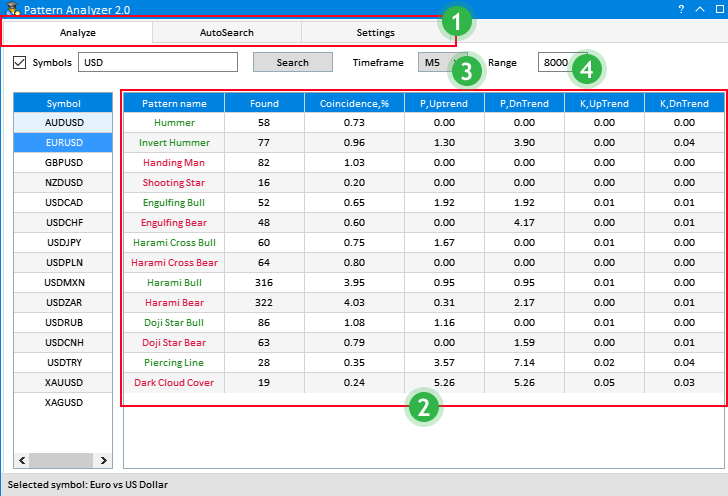Fig.1 Interface of the Analyze tab in the previous version

Point 1. Tab arrangement and dimensions.

In Fig.1, tabs marked by 1 are located in the upper part of the window. The upper right part here is empty and is not used, however the part is not enough to add more tabs. The text font is too small. These three tabs have been moved to the left part of the window: they are now arranged vertically and are more visible. Moreover, there is additional space to add more sections.

Point 2. Tables with pattern testing results.

The visual data presentation is not very efficient. Therefore, the font, the row height and the table size have been increased for better readability.

Point 3. Current timeframe selection.

The selection structure 'Timeframe -> Result' for all patterns limits the visual display of testing results. To improve this, we will develop a multi-timeframe selection option, as well as an individual selection of analyzed patterns. This will allow a more flexible customization of operation with patterns.

Point 4. Sampling range.

The idea implemented in the previous version was to test in the range from the current data to a certain number of candlesticks in history. A more specific selection from one date to another was not available. Therefore the range selection method will be revised. Fig.2 below features the solution of all the above mentioned issues and possible improvements.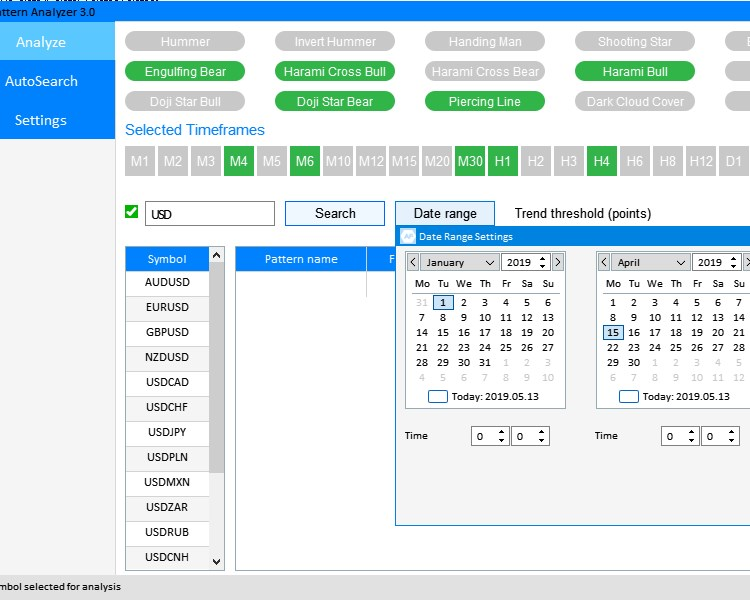Fig. 2. Updated interface of the Analyze tab.

Here are the solutions to the above points.

• Vertical arrangement of tabs in the left part of the window.
• Manual selection of analyzed patterns.
• Manual selection of current timeframes.
• A new 'Date range' tool for the testing range instead of using the number of candlesticks.

The application window has become larger to display new elements. Another important new feature is that the 'Trend threshold value' parameter (Fig.3) in points has been moved from the Settings tab to both Analyze and AutoSearch tabs. The setting is individual for each of the tabs.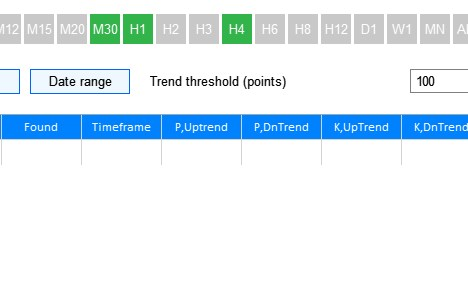Fig.3 New position of the Trend Threshold parameter

The last element which has been changed is the structure of the Results table. The occurrence column has been removed and a more relevant Timeframe parameter has been added instead.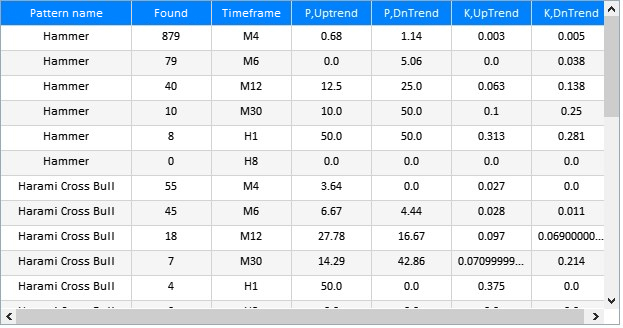Fig. 4. The new structure of the results table

Now let's look at improvements in the second tab, AutoSearch, which works with generated patterns.

Point 1. Settings in different tabs.

Settings directly related to the AutoSearch section were located in Setting tab, so one needed to constantly switch between the tabs AutoSearch and Setting in order to change the settings. That is why almost all settings have been moved to the AutoSearch tab. Further improvements have been implemented in relation to the Threshold trend value, selection of current timeframes and the date range. The result of AutoSearch tab update is shown in Fig.5.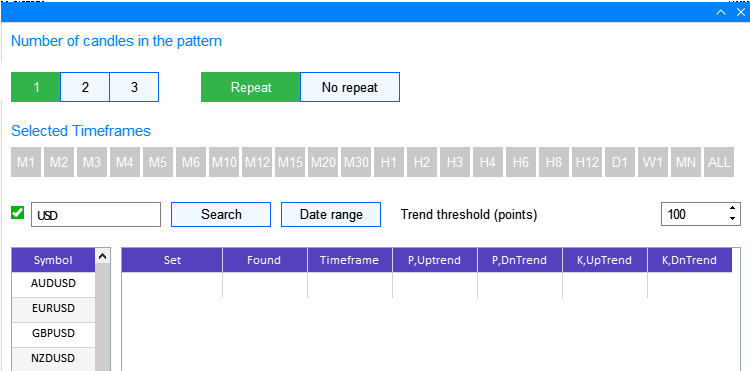Fig.5 Updated functionality in the AutoSearch tab

This enables a more convenient work with patterns. The date range in this tab is also individual.

Let us consider in more detail the implementation of the above updates, as well as changes in calculations.

Structure of application windows. Main window creation method.

The CProgram::CreateGUI() method, which is responsible for the graphical interface creation has been supplemented:

```//+------------------------------------------------------------------+
//| Creates the graphical interface of the program                   |
//+------------------------------------------------------------------+
bool CProgram::CreateGUI(void)
{
//--- Creating a panel
if(!CreateWindow("Pattern Analyzer"))
return(false);
//--- Creating a dialog window
if(!CreateWindowSetting1("Settings"))
return(false);
//--- Creating a dialog window
if(!CreateWindowSetting2("Date range settings"))
return(false);
//--- Creating a dialog window
if(!CreateWindowSetting3("Date range settings"))
return(false);
//--- Complete GUI creation
CWndEvents::CompletedGUI();
return(true);
}
//+-----------------------------------------------------------------
```

CreateWindowSetting2() and CreateWindowSetting3() are responsible for the display of the new date range selection tool shown in Fig.1. The main app window creation method CreateWindow() has also been redesigned. It has been divided into three blocks corresponding to the UI elements of each of the tabs: Analyze, AutoSearch and Setting.

```//+------------------------------------------------------------------+
//| The Analyze tab                                                  |
//+------------------------------------------------------------------+
//--- Create buttons of the pattern set
if(!CreatePatternSet(m_patterns,10,10))
return(false);
if(!CreateTFLabel(m_text_labels,10,100,0))
return(false);
//--- Create buttons of the timeframe set
if(!CreateTimeframeSet(m_timeframes,10,125,0))
return(false);
//--- Symbol filter search window
if(!CreateSymbolsFilter(m_symb_filter1,m_request1,10,180,0))
return(false);
//--- Create a button for date range selection
if(!CreateDateRange(m_request3,280,180,0))
return(false);
//--- Create an entry field for the threshold profit value
if(!CreateThresholdValue(m_threshold1,400,180,100,0))
return(false);
//--- Create a table of symbols
if(!CreateSymbTable(m_symb_table1,10,225,0))
return(false);
//--- Create a table of results
if(!CreateTable1(m_table1,120,225,0))
return(false);
```

The methods for displaying new interface elements have been added in the first tab.

• CreatePatternSet(). A new method which displays a set of switchable pattern selection buttons.
• CreateTFLabel(). Text leader label for a set of timeframes.
• CreateTimeframeSet(). A set of switchable timeframe buttons.
• CreateDateRange(). A new button, which, when clicked, opens the dialog box for selecting the date range for the analysis.
• CreateThresholdValue(). A revised method which displays the Trend threshold value in points (Fig.3).

```//+------------------------------------------------------------------+
//| The AutoSearch tab                                               |
//+------------------------------------------------------------------+
if(!CreateTFLabel(m_text_labels,10,10,1))
return(false);
//--- Buttons
if(!CreateDualButton(m_buttons,m_buttons,200,50))
return(false);
if(!CreateTripleButton(m_buttons,m_buttons,m_buttons,10,50))
return(false);
if(!CreateTFLabel(m_text_labels,10,100,1))
return(false);
//--- Create buttons of the timeframe set
if(!CreateTimeframeSet(m_timeframes1,10,125,1))
return(false);
//--- Edit fields
if(!CreateSymbolsFilter(m_symb_filter2,m_request2,10,180,1))
return(false);
//--- Create a button for date range selection
if(!CreateDateRange(m_request4,280,180,1))
return(false);
//--- Create an entry field for the threshold profit value
if(!CreateThresholdValue(m_threshold2,400,180,100,1))
return(false);
//--- Create a table of symbols
if(!CreateSymbTable(m_symb_table2,10,225,1))
return(false);
//--- Create a table of results
if(!CreateTable2(m_table2,120,225,1))
return(false);
```

Also the following methods have been moved to the second AutoSearch tab from the Setting tab (Fig.5): methods responsible for the display of elements related to the selection of generated pattern sizes CreateTripleButton() and the switchable option Repeat/No repeat with the CreateDualButton() method. New methods have been added: methods responsible for the timeframe header and their selection.

```//+------------------------------------------------------------------+
//| The Settings tab                                                 |
//+------------------------------------------------------------------+
//--- Creating candlestick settings
if(!CreateCandle(m_pictures,m_buttons,m_candle_names,"Long",10,10,"Images\\EasyAndFastGUI\\Candles\\long.bmp"))
return(false);
if(!CreateCandle(m_pictures,m_buttons,m_candle_names,"Short",104,10,"Images\\EasyAndFastGUI\\Candles\\short.bmp"))
return(false);
if(!CreateCandle(m_pictures,m_buttons,m_candle_names,"Spinning top",198,10,"Images\\EasyAndFastGUI\\Candles\\spin.bmp"))
return(false);
if(!CreateCandle(m_pictures,m_buttons,m_candle_names,"Doji",292,10,"Images\\EasyAndFastGUI\\Candles\\doji.bmp"))
return(false);
if(!CreateCandle(m_pictures,m_buttons,m_candle_names,"Marubozu",386,10,"Images\\EasyAndFastGUI\\Candles\\maribozu.bmp"))
return(false);
if(!CreateCandle(m_pictures,m_buttons,m_candle_names,"Hammer",480,10,"Images\\EasyAndFastGUI\\Candles\\hammer.bmp"))
return(false);
//--- Text labels
if(!CreateTextLabel(m_text_labels,10,140))
return(false);
if(!CreateTextLabel(m_text_labels,300,140))
return(false);
//--- Edit fields
if(!CreateCoef(m_coef1,10,180,"K1",1))
return(false);
if(!CreateCoef(m_coef2,100,180,"K2",0.5))
return(false);
if(!CreateCoef(m_coef3,200,180,"K3",0.25))
return(false);
if(!CreateLanguageSetting(m_lang_setting,10,240,2))
return(false);
//--- List views
if(!CreateListView(300,180))
return(false);
//---
if(!CreateCheckBox(m_checkbox1,300+8,160,"All candlesticks"))
return(false);
//--- Status Bar
if(!CreateStatusBar(1,26))
return(false);
```

The Setting section now contains less elements. It contains individual candlestick settings, settings of ratios for the calculation of probability and efficiency coefficients, interface language selection and candlestick selection for pattern generation in the AutoSearch tab. Next, consider the new methods in more detail.

Method for creating pattern selection CreatePatternSet(). It is a set of switchable buttons for selecting existing patterns for analysis.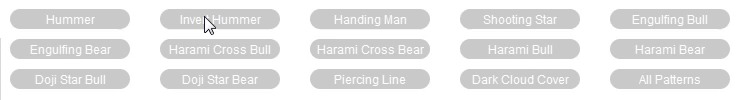Fig.6 The principle of pattern selector for analysis

The implementation is presented below:

```//+------------------------------------------------------------------+
//| Creates a set of pattern buttons                                 |
//+------------------------------------------------------------------+
bool CProgram::CreatePatternSet(CButton &button[],int x_gap,int y_gap)
{
ArrayResize(button,15);
string pattern_names=
{
"Hummer",
"Invert Hummer",
"Handing Man",
"Shooting Star",
"Engulfing Bull",
"Engulfing Bear",
"Harami Cross Bull",
"Harami Cross Bear",
"Harami Bull",
"Harami Bear",
"Doji Star Bull",
"Doji Star Bear",
"Piercing Line",
"Dark Cloud Cover",
"All Patterns"
};
int k1=x_gap,k2=x_gap,k3=x_gap;
for(int i=0;i<=14;i++)
{
if(i<5)
{
CreatePatternButton(button[i],pattern_names[i],k1,y_gap);
k1+=150;
}
else if(i>=5 && i<10)
{
CreatePatternButton(button[i],pattern_names[i],k2,y_gap+30);
k2+=150;
}
else if(i>=10 && i<14)
{
CreatePatternButton(button[i],pattern_names[i],k3,y_gap+60);
k3+=150;
}
else if(i==14)
{
CreatePatternButton(button[i],pattern_names[i],k3,y_gap+60);
}
}
return(true);
}
//+------------------------------------------------------------------+
//| Creates a button for selecting a pattern for analysis            |
//+------------------------------------------------------------------+
#resource "\\Images\\EasyAndFastGUI\\Candles\\passive.bmp"
#resource "\\Images\\EasyAndFastGUI\\Candles\\pressed.bmp"
//+------------------------------------------------------------------+
//|                                                                  |
//+------------------------------------------------------------------+
bool CProgram::CreatePatternButton(CButton &button,const string candlename,const int x_gap,const int y_gap)
{
//--- Save the pointer to the main control
button.MainPointer(m_tabs1);
//--- Attach to tab
//--- Properties
button.XSize(120);
button.YSize(20);
button.Font("Trebuchet");
button.FontSize(9);
button.LabelColor(clrWhite);
button.LabelColorHover(clrWhite);
button.LabelColorPressed(clrWhite);
button.IsCenterText(true);
button.TwoState(true);
button.IconFile("Images\\EasyAndFastGUI\\Candles\\passive.bmp");
button.IconFilePressed("Images\\EasyAndFastGUI\\Candles\\pressed.bmp");
//--- Create a control
if(!button.CreateButton(candlename,x_gap,y_gap))
return(false);
//--- Add the element pointer to the data base
return(true);
}
```

Pay attention to the last button 'All Patterns' which selects/unselects all patterns. The button press is processed by additional code in the button press event handing section:

```   if(id==CHARTEVENT_CUSTOM+ON_CLICK_BUTTON)
{
//--- Select and unselect all pattern buttons
if(lparam==m_patterns.Id())
{
if(m_patterns.IsPressed())
{
for(int i=0;i<14;i++)
m_patterns[i].IsPressed(true);
}
else if(!m_patterns.IsPressed())
{
for(int i=0;i<14;i++)
m_patterns[i].IsPressed(false);
}
for(int i=0;i<14;i++)
m_patterns[i].Update(true);
}
...
}
```

The current timeframe selection method CreateTimeframeSet() is very similar to the previous one. It also has a set of switchable buttons which select timeframes for analysis.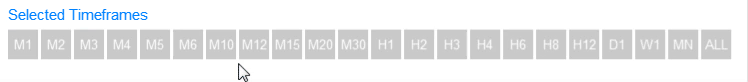Fig.7 The principle of timeframe selector for analysis

The implementation is presented in the below code:

```//+------------------------------------------------------------------+
//| Creates a set of timeframe buttons                               |
//+------------------------------------------------------------------+
bool CProgram::CreateTimeframeSet(CButton &button[],int x_gap,int y_gap,const int tab)
{
ArrayResize(button,22);
string timeframe_names=
{"M1","M2","M3","M4","M5","M6","M10","M12","M15","M20","M30","H1","H2","H3","H4","H6","H8","H12","D1","W1","MN","ALL"};
int k1=x_gap,k2=x_gap;
for(int i=0;i<22;i++)
{
CreateTimeframeButton(button[i],timeframe_names[i],k1,y_gap,tab);
k1+=33;
}
return(true);
}
//+------------------------------------------------------------------+
//|                                                                  |
//+------------------------------------------------------------------+
bool CProgram::CreateTimeframeButton(CButton &button,const string candlename,const int x_gap,const int y_gap,const int tab)
{
//--- Save the pointer to the main control
button.MainPointer(m_tabs1);
//--- Attach to tab
//--- Properties
button.XSize(30);
button.YSize(30);
button.Font("Trebuchet");
button.FontSize(10);
button.LabelColor(clrWhite);
button.LabelColorHover(clrWhite);
button.LabelColorPressed(clrWhite);
button.BackColor(C'200,200,200');
button.BackColorHover(C'200,200,200');
button.BackColorPressed(C'50,180,75');
button.BorderColor(C'200,200,200');
button.BorderColorHover(C'200,200,200');
button.BorderColorPressed(C'50,180,75');
button.IsCenterText(true);
button.TwoState(true);
//--- Create a control
if(!button.CreateButton(candlename,x_gap,y_gap))
return(false);
//--- Add the element pointer to the data base
return(true);
}
```

It also has a button for selecting/unselecting all timeframes and is processed in the button-press handling section:

```//--- Select and unselect all pattern buttons
if(lparam==m_timeframes.Id())
{
if(m_timeframes.IsPressed())
{
for(int i=0;i<21;i++)
m_timeframes[i].IsPressed(true);
}
else if(!m_timeframes.IsPressed())
{
for(int i=0;i<21;i++)
m_timeframes[i].IsPressed(false);
}
for(int i=0;i<21;i++)
m_timeframes[i].Update(true);
}
```

The next new item is the Date Range button. It is part of the new composite sample range setting tool. It is implemented using the CreateDateRange() method.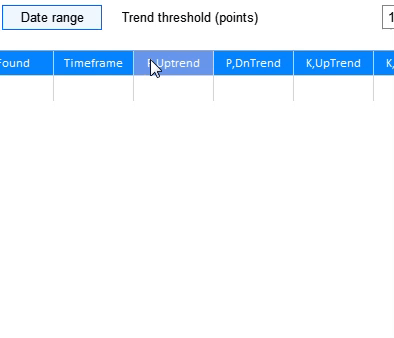Fig.8 The principle of date range selector for analysis

The implementation is presented below:

```//+------------------------------------------------------------------+
//| Creates a button to show the date range selection window         |
//+------------------------------------------------------------------+
bool CProgram::CreateDateRange(CButton &button,const int x_gap,const int y_gap,const int tab)
{
//--- Save the pointer to the main control
button.MainPointer(m_tabs1);
//--- Attach to tab
//--- Properties
button.XSize(100);
button.YSize(25);
button.Font("Trebuchet");
button.FontSize(10);
button.IsHighlighted(false);
button.IsCenterText(true);
button.BorderColor(C'0,100,255');
button.BackColor(clrAliceBlue);
//--- Create a control
if(!button.CreateButton("",x_gap,y_gap))
return(false);
//--- Add the element pointer to the data base
return(true);
}
```

The button press event handler also includes code which is responsible for the display of the dialog box with the date range:

```      //---
if(lparam==m_request3.Id())
{
int x=m_request3.X();
int y=m_request3.Y()+m_request3.YSize();
m_window.X(x);
m_window.Y(y);
m_window.OpenWindow();
val=(m_lang_index==0)?"Настройки диапазона дат":"Date Range Settings";
m_window.LabelText(val);
}
```

There is no need to describe new elements which have been added in the tab, since they are similar to the implementation of the Analyze tab elements, with the exception of coordinate parameters. Therefore, let's consider methods responsible for the display of other new windows.

Structure of application windows. A method for creating the application dialog box.

The methods which display dialog boxes for the Analyze and AutoSearch tabs are similar and thus we will consider one of them.

```//+------------------------------------------------------------------+
//| Creates a date range selection dialog in the Analyze tab         |
//+------------------------------------------------------------------+
bool CProgram::CreateWindowSetting2(const string caption_text)
{
//--- Add the pointer to the window array
//--- Coordinates
int x=m_request3.X();
int y=m_request3.Y()+m_request3.YSize();
//--- Properties
m_window.XSize(372);
m_window.YSize(300);
m_window.WindowType(W_DIALOG);

//--- Create the form
if(!m_window.CreateWindow(m_chart_id,m_subwin,caption_text,x,y))
return(false);
//---
if(!CreateCalendar(m_calendar1,m_window,10,25,D'01.01.2018',2))
return(false);
if(!CreateCalendar(m_calendar2,m_window,201,25,m_calendar2.Today(),2))
return(false);
//---
if(!CreateTimeEdit(m_time_edit1,m_window,10,200,"Time",2))
return(false);
if(!CreateTimeEdit(m_time_edit2,m_window,200,200,"Time",2))
return(false);
//---
return(true);
}
```

Calculation part. Redesigned candlestick and pattern finder methods.

Due to serious changes in the user interface structure, as well as due to addition of new elements and deletion of some old ones, the calculation methods have also changed. Two calculation methods are available in the current application: the first one is used for existing patterns and the second method is used for generated patterns.

Calculation is launched after a click on one of the available trading instruments in the Symbols table. This rule applies to both tabs, Analyze and AutoSearch. One of the two methods is called depending on the tab.

```//+------------------------------------------------------------------+
//| Symbol change in the Analyze tab                                 |
//+------------------------------------------------------------------+
bool CProgram::ChangeSymbol1(const long id)
{
//--- Check the element ID
if(id!=m_symb_table1.Id())
return(false);
//--- Exit if the line is not selected
if(m_symb_table1.SelectedItem()==WRONG_VALUE)
{
//--- Show the full symbol description in the status bar
m_status_bar.SetValue(0,"No symbol selected for analysis");
m_status_bar.GetItemPointer(0).Update(true);
return(false);
}
//--- Get a symbol
string symbol=m_symb_table1.GetValue(0,m_symb_table1.SelectedItem());
//--- Show the full symbol description in the status bar
string val=(m_lang_index==0)?"Выбранный символ: ":"Selected symbol: ";
m_status_bar.SetValue(0,val+::SymbolInfoString(symbol,SYMBOL_DESCRIPTION));
m_status_bar.GetItemPointer(0).Update(true);
//---
GetPatternType(symbol);
return(true);
}
//+------------------------------------------------------------------+
//| Symbol change in the AutoSearch tab                              |
//+------------------------------------------------------------------+
bool CProgram::ChangeSymbol2(const long id)
{
//--- Check the element ID
if(id!=m_symb_table2.Id())
return(false);
//--- Exit if the line is not selected
if(m_symb_table2.SelectedItem()==WRONG_VALUE)
{
//--- Show the full symbol description in the status bar
m_status_bar.SetValue(0,"No symbol selected for analysis");
m_status_bar.GetItemPointer(0).Update(true);
return(false);
}
//--- Get a symbol
string symbol=m_symb_table2.GetValue(0,m_symb_table2.SelectedItem());
//--- Show the full symbol description in the status bar
string val=(m_lang_index==0)?"Выбранный символ: ":"Selected symbol: ";
m_status_bar.SetValue(0,val+::SymbolInfoString(symbol,SYMBOL_DESCRIPTION));
m_status_bar.GetItemPointer(0).Update(true);
//---
if(!GetCandleCombitation())
{
if(m_lang_index==0)
MessageBox("Число выбранных свечей меньше размера исследуемого паттерна!","Ошибка",MB_OK);
else if(m_lang_index==1)
MessageBox("The number of selected candles is less than the size of the studied pattern!","Error",MB_OK);
return(false);
}
//---
GetPatternType(symbol,m_total_combination);
return(true);
}
```

The GetPattertType() method with two different argument types is called at the end of each method. This is the key method in search for patterns and in handling of obtained results. Now let's consider each of the method in detail.

The first method type is used to search for existing patterns.

`   bool              GetPatternType(const string symbol);`

The method has quite a lengthy implementation, therefore an example for only one pattern will be provided here.

```//+------------------------------------------------------------------+
//| Pattern recognition                                              |
//+------------------------------------------------------------------+
bool CProgram::GetPatternType(const string symbol)
{
CANDLE_STRUCTURE cand1,cand2;
//---
RATING_SET hummer_coef[];
RATING_SET invert_hummer_coef[];
RATING_SET handing_man_coef[];
RATING_SET shooting_star_coef[];
RATING_SET engulfing_bull_coef[];
RATING_SET engulfing_bear_coef[];
RATING_SET harami_cross_bull_coef[];
RATING_SET harami_cross_bear_coef[];
RATING_SET harami_bull_coef[];
RATING_SET harami_bear_coef[];
RATING_SET doji_star_bull_coef[];
RATING_SET doji_star_bear_coef[];
RATING_SET piercing_line_coef[];
RATING_SET dark_cloud_cover_coef[];
//--- Receive data for selected timeframes
GetTimeframes(m_timeframes,m_cur_timeframes1);
int total=ArraySize(m_cur_timeframes1);
//--- Check at least one selected timerame
if(total<1)
{
if(m_lang_index==0)
MessageBox("Вы не выбрали рабочий таймфрейм!","Ошибка",MB_OK);
else if(m_lang_index==1)
MessageBox("You have not selected a working timeframe!","Error",MB_OK);
return(false);
}
int count=0;
m_total_row=0;
m_table_number=1;
//--- Delete all rows
m_table1.DeleteAllRows();
//--- Get the date range
datetime start=StringToTime(TimeToString(m_calendar1.SelectedDate(),TIME_DATE)+" "+(string)m_time_edit1.GetHours()+":"+(string)m_time_edit1.GetMinutes()+":00");
datetime end=StringToTime(TimeToString(m_calendar2.SelectedDate(),TIME_DATE)+" "+(string)m_time_edit2.GetHours()+":"+(string)m_time_edit2.GetMinutes()+":00");
//--- Check specified dates
if(start>end || end>TimeCurrent())
{
if(m_lang_index==0)
MessageBox("Неправильно выбран диапазон дат!","Ошибка",MB_OK);
else if(m_lang_index==1)
MessageBox("Incorrect date range selected!","Error",MB_OK);
return(false);
}
//--- Hammer, bullish model
if(m_patterns.IsPressed())
{
ArrayResize(m_hummer_total,total);
ArrayResize(hummer_coef,total);
ZeroMemory(m_hummer_total);
ZeroMemory(hummer_coef);
ZeroMemory(cand1);
count++;
//--- Calculation by timeframes
for(int j=0;j<total;j++)
{
MqlRates rt[];
ZeroMemory(rt);
int copied=CopyRates(symbol,m_cur_timeframes1[j],start,end,rt);
for(int i=0;i<copied;i++)
{
GetCandleType(symbol,cand1,m_cur_timeframes1[j],i);             // Current candlestick
if(cand1.trend==DOWN &&                                        // Checking the trend direction
cand1.type==CAND_HAMMER)                                    // Checking the "Hammer"
{
m_hummer_total[j]++;
GetCategory(symbol,i+3,hummer_coef[j],m_cur_timeframes1[j],m_threshold_value1);
}
}
}
}
...
//---
if(count>0)
{
//---
m_table1.DeleteRow(m_total_row);
//--- Update the table
m_table1.Update(true);
m_table1.GetScrollVPointer().Update(true);
}
else
{
if(m_lang_index==0)
MessageBox("Вы не выбрали паттерн!","Ошибка",MB_OK);
else if(m_lang_index==1)
MessageBox("You have not chosen a pattern!","Error",MB_OK);
}
return(true);
}
```

The operation algorithm is performed as follows:

• Structures required for calculation are updated.
• Use the  GetTimeframes() method to obtain from the UI data about selected timeframes.
• Process a possible error when no timeframe is selected.
• If at least one timeframe is selected, get the data of the date range and check if the inputs are correct. Also there should be no input errors.
• Then check if every of possible patterns is selected for calculation and search for that pattern in the earlier selected timeframes and range.
• At the end check if there is at least one selected pattern for the calculation. If a pattern is selected, output the results table. If no pattern is selected, print the appropriate message.

Next, we consider the methods which existed in earlier versions and were changed in the updated version, as well as one new method for the calculation of obtained data and their output in the results table:

• Specified candlestick type search method GetCandleType().
• Found pattern efficiency evaluation method GetCategory().
• Method for calculating obtained pattern data and outputting results to the table AddRow().

The GetPatternType() method for pattern search has two different implementations, while these three methods are universal. Let's consider them in detail:

```//+------------------------------------------------------------------+
//| Candlestick type recognition                                     |
//+------------------------------------------------------------------+
bool CProgram::GetCandleType(const string symbol,CANDLE_STRUCTURE &res,ENUM_TIMEFRAMES timeframe,const int shift)
{
MqlRates rt[];
int aver_period=5;
double aver=0.0;
datetime start=TimeCurrent();
SymbolSelect(symbol,true);
//--- Get the start date from the range depending on the type of patterns
if(m_table_number==1)
start=StringToTime(TimeToString(m_calendar1.SelectedDate(),TIME_DATE)+" "+(string)m_time_edit1.GetHours()+":"+(string)m_time_edit1.GetMinutes()+":00");
else if(m_table_number==2)
start=StringToTime(TimeToString(m_calendar3.SelectedDate(),TIME_DATE)+" "+(string)m_time_edit3.GetHours()+":"+(string)m_time_edit3.GetMinutes()+":00");
//--- Shift date
start+=PeriodSeconds(timeframe)*shift;
int copied=CopyRates(symbol,timeframe,start,aver_period+1,rt);
if(copied<6)
{
Print(start,": Not enough data for calculation — ",GetLastError());
}
//--- Get details of the previous candlestick
if(copied<aver_period)
return(false);
//---
res.open=rt[aver_period].open;
res.high=rt[aver_period].high;
res.low=rt[aver_period].low;
res.close=rt[aver_period].close;
//--- Determine the trend direction
for(int i=0;i<aver_period;i++)
aver+=rt[i].close;

aver/=aver_period;

if(aver<res.close)
res.trend=UPPER;
if(aver>res.close)
res.trend=DOWN;
if(aver==res.close)
res.trend=FLAT;
//--- Determine if it is a bullish or a bearish candlestick
res.bull=res.open<res.close;
//--- Get the absolute size of candlestick body
res.bodysize=MathAbs(res.open-res.close);
if(res.bull)
{
}
double HL=res.high-res.low;
//--- Calculate average body size of previous candlesticks
double sum=0;
for(int i=1; i<=aver_period; i++)
sum+=MathAbs(rt[i].open-rt[i].close);
sum/=aver_period;

//--- Determine the candlestick type
res.type=CAND_NONE;
//--- long
if(res.bodysize>sum*m_long_coef && res.bull)
res.type=CAND_LONG_BULL;
//--- sort
if(res.bodysize<sum*m_short_coef && res.bull)
res.type=CAND_SHORT_BULL;
//--- long bear
if(res.bodysize>sum*m_long_coef && !res.bull)
res.type=CAND_LONG_BEAR;
//--- sort bear
if(res.bodysize<sum*m_short_coef && !res.bull)
res.type=CAND_SHORT_BEAR;
//--- doji
if(res.bodysize<HL*m_doji_coef)
res.type=CAND_DOJI;
//--- marubozu
res.type=CAND_MARIBOZU;
//--- hammer
res.type=CAND_HAMMER;
//--- invert hammer
res.type=CAND_INVERT_HAMMER;
//--- spinning top
res.type=CAND_SPIN_TOP;
//---
ArrayFree(rt);
return(true);
}
```

The method algorithm is as follows: get the initial date from the selection range depending on the patterns you want to analyze, existing or generated ones. Since this method is used in a calculation cycle in the date range, change the initial date by shifting it from past towards the future by one candlestick of the given timeframe. Then copy data required for the calculation of simple candlestick types. If this data is not enough, display an appropriate message to the user.

Important note! It is necessary to monitor the availability of historical data in the MetaTrader 5 terminal, otherwise the application may not work correctly.

If data is enough, a check is performed of whether the current candlestick belongs to a simple candlestick type.

As is known from previous articles, the GetCategory() method checks the price behavior after pattern occurrence using historic data.

```//+------------------------------------------------------------------+
//| Determine profit categories                                      |
//+------------------------------------------------------------------+
bool CProgram::GetCategory(const string symbol,const int shift,RATING_SET &rate,ENUM_TIMEFRAMES timeframe,int threshold)
{
MqlRates rt[];
datetime start=TimeCurrent();
if(m_table_number==1)
start=StringToTime(TimeToString(m_calendar1.SelectedDate(),TIME_DATE)+" "+(string)m_time_edit1.GetHours()+":"+(string)m_time_edit1.GetMinutes()+":00");
else if(m_table_number==2)
start=StringToTime(TimeToString(m_calendar3.SelectedDate(),TIME_DATE)+" "+(string)m_time_edit3.GetHours()+":"+(string)m_time_edit3.GetMinutes()+":00");
start+=PeriodSeconds(timeframe)*shift;
int copied=CopyRates(symbol,timeframe,start,4,rt);
//--- Get details of the previous candlestick
if(copied<4)
{
return(false);
}
double high1,high2,high3,low1,low2,low3,close0,point;
close0=rt.close;
high1=rt.high;
high2=rt.high;
high3=rt.high;
low1=rt.low;
low2=rt.low;
low3=rt.low;
if(!SymbolInfoDouble(symbol,SYMBOL_POINT,point))
return(false);

//--- Check if it is the Uptrend
if((int)((high1-close0)/point)>=threshold)
{
rate.a_uptrend++;
}
else if((int)((high2-close0)/point)>=threshold)
{
rate.b_uptrend++;
}
else if((int)((high3-close0)/point)>=threshold)
{
rate.c_uptrend++;
}

//--- Check if it is the Downtrend
if((int)((close0-low1)/point)>=threshold)
{
rate.a_dntrend++;
}
else if((int)((close0-low2)/point)>=threshold)
{
rate.b_dntrend++;
}
else if((int)((close0-low3)/point)>=threshold)
{
rate.c_dntrend++;
}
return(true);
}
```

In this method algorithm only the method for obtaining data about analyzed candlesticks has changed. This is directly related to the new time range selection tool.

The last common method for both GetPatternType() is getting, calculating and displaying data in the results table.

```//+------------------------------------------------------------------+
//| Get, calculate and display data in the results table             |
//+------------------------------------------------------------------+
void CProgram::AddRow(CTable &table,string pattern_name,RATING_SET &rate,int found,ENUM_TIMEFRAMES timeframe)
{
int row=m_total_row;
int total_patterns=ArraySize(m_total_combination);
double p1,p2,k1,k2;
int sum1=0,sum2=0;
sum1=rate.a_uptrend+rate.b_uptrend+rate.c_uptrend;
sum2=rate.a_dntrend+rate.b_dntrend+rate.c_dntrend;
//---
p1=(found>0)?NormalizeDouble((double)sum1/found*100,2):0;
p2=(found>0)?NormalizeDouble((double)sum2/found*100,2):0;
k1=(found>0)?NormalizeDouble((m_k1*rate.a_uptrend+m_k2*rate.b_uptrend+m_k3*rate.c_uptrend)/found,3):0;
k2=(found>0)?NormalizeDouble((m_k1*rate.a_dntrend+m_k2*rate.b_dntrend+m_k3*rate.c_dntrend)/found,3):0;

//---
if(m_table_number==1)
table.SetValue(0,row,pattern_name);
else if(m_table_number==2)
{
if(row<total_patterns)
table.SetValue(0,row,m_total_combination[row]);
else if(row>=total_patterns)
{
int i=row-int(total_patterns*MathFloor(double(row)/total_patterns));
table.SetValue(0,row,m_total_combination[i]);
}
}
table.SetValue(1,row,(string)found);
table.SetValue(2,row,TimeframeToString(timeframe));
table.SetValue(3,row,(string)p1,2);
table.SetValue(4,row,(string)p2,2);
table.SetValue(5,row,(string)k1,2);
table.SetValue(6,row,(string)k2,2);
ZeroMemory(rate);
m_total_row++;
}
//+------------------------------------------------------------------+
```

The method receives all data for calculation in its arguments. Data handling algorithm is quite simple. Two moments should be mentioned here. In previous application versions, the exact number of rows was known in advance. For example, when processing existing pattern data, the results table always had the same number of rows equal to the number of preset patterns, i.e. 14. Now, the user can choose any number of patterns or working timeframes and thus the number of rows is not known. Therefore a simple row counter m_total_row was added. The call of the AddRow() method adds a row to the results table based on two signs: pattern and timeframe.

The second point concerns the AutoSearch tab. In previous versions, the finite number of rows was equal to the number of combinations of generated patterns. The previous algorithm is not suitable now for the same reason: the number of timeframes is unknown. Therefore, the entire array of generated combinations should be written again for each of the selected timeframes.

Let's consider the second variant of the GetPatternType() method.

`bool              GetPatternType(const string symbol,string &total_combination[]);`

Here, in addition to the current symbol, the second parameter is the link to the string array of generated patterns.

```//+------------------------------------------------------------------+
//|                                                                  |
//+------------------------------------------------------------------+
bool CProgram::GetPatternType(const string symbol,string &total_combination[])
{
CANDLE_STRUCTURE cand1[],cand2[],cand3[],cur_cand,prev_cand,prev_cand2;
RATING_SET ratings;
int total_patterns,m_pattern_total[];
string elements[];
//---
total_patterns=ArraySize(total_combination);
ArrayResize(cand1,total_patterns);
ArrayResize(cand2,total_patterns);
ArrayResize(cand3,total_patterns);
ArrayResize(m_pattern_total,total_patterns);
ArrayResize(elements,m_pattern_size);
//---
for(int i=0;i<total_patterns;i++)
{
StringReplace(total_combination[i],"[","");
StringReplace(total_combination[i],"]","");
if(m_pattern_size>1)
{
ushort sep=StringGetCharacter(",",0);
StringSplit(total_combination[i],sep,elements);
}
m_pattern_total[i]=0;
if(m_pattern_size==1)
IndexToPatternType(cand1[i],(int)total_combination[i]);
else if(m_pattern_size==2)
{
IndexToPatternType(cand1[i],(int)elements);
IndexToPatternType(cand2[i],(int)elements);
}
else if(m_pattern_size==3)
{
IndexToPatternType(cand1[i],(int)elements);
IndexToPatternType(cand2[i],(int)elements);
IndexToPatternType(cand3[i],(int)elements);
}
}
//---
GetTimeframes(m_timeframes1,m_cur_timeframes2);
int total=ArraySize(m_cur_timeframes2);
if(total<1)
{
if(m_lang_index==0)
MessageBox("Вы не выбрали рабочий таймфрейм!","Ошибка",MB_OK);
else if(m_lang_index==1)
MessageBox("You have not selected a working timeframe!","Error",MB_OK);
return(false);
}
m_total_row=0;
m_table_number=2;
//--- Delete all rows
m_table2.DeleteAllRows();
//---
datetime start=StringToTime(TimeToString(m_calendar3.SelectedDate(),TIME_DATE)+" "+(string)m_time_edit3.GetHours()+":"+(string)m_time_edit3.GetMinutes()+":00");
datetime end=StringToTime(TimeToString(m_calendar4.SelectedDate(),TIME_DATE)+" "+(string)m_time_edit4.GetHours()+":"+(string)m_time_edit4.GetMinutes()+":00");
//---
if(start>end || end>TimeCurrent())
{
if(m_lang_index==0)
MessageBox("Неправильно выбран диапазон дат!","Ошибка",MB_OK);
else if(m_lang_index==1)
MessageBox("Incorrect date range selected!","Error",MB_OK);
return(false);
}
//---
if(m_pattern_size==1)
{
ZeroMemory(cur_cand);
//--- Calculation by timeframes
for(int i=0;i<total;i++)
{
MqlRates rt[];
ZeroMemory(rt);
ZeroMemory(ratings);
int copied=CopyRates(symbol,m_cur_timeframes2[i],start,end,rt);
//--- Calculation by patterns
for(int j=0;j<total_patterns;j++)
{
//--- Calculation by a date range
for(int k=0;k<copied;k++)
{
//--- Get the current candlestick type
GetCandleType(symbol,cur_cand,m_cur_timeframes2[i],k);                 // current candlestick
//---
if(cur_cand.type==cand1[j].type && cur_cand.bull==cand1[j].bull)
{
m_pattern_total[j]++;
GetCategory(symbol,k+3,ratings,m_cur_timeframes2[i],m_threshold_value2);
}
}
m_pattern_total[j]=0;
}
}
}
else if(m_pattern_size==2)
{
ZeroMemory(cur_cand);
ZeroMemory(prev_cand);
//--- Calculation by timeframes
for(int i=0;i<total;i++)
{
MqlRates rt[];
ZeroMemory(rt);
ZeroMemory(ratings);
int copied=CopyRates(symbol,m_cur_timeframes2[i],start,end,rt);
//--- Calculation by patterns
for(int j=0;j<total_patterns;j++)
{
//--- Calculation by a date range
for(int k=0;k<copied;k++)
{
//--- Get the current candlestick type
GetCandleType(symbol,prev_cand,m_cur_timeframes2[i],k+1);               // previous candlestick
GetCandleType(symbol,cur_cand,m_cur_timeframes2[i],k);                  // current candlestick
//---
if(cur_cand.type==cand1[j].type && cur_cand.bull==cand1[j].bull &&
prev_cand.type==cand2[j].type && prev_cand.bull==cand2[j].bull)
{
m_pattern_total[j]++;
GetCategory(symbol,k+4,ratings,m_cur_timeframes2[i],m_threshold_value2);
}
}
m_pattern_total[j]=0;
}
}
}
else if(m_pattern_size==3)
{
ZeroMemory(cur_cand);
ZeroMemory(prev_cand);
ZeroMemory(prev_cand2);
//--- Calculation by timeframes
for(int i=0;i<total;i++)
{
MqlRates rt[];
ZeroMemory(ratings);
int copied=CopyRates(symbol,m_cur_timeframes2[i],start,end,rt);
//--- Calculation by patterns
for(int j=0;j<total_patterns;j++)
{
//--- Calculation by a date range
for(int k=0;k<copied;k++)
{
//--- Get the current candlestick type
GetCandleType(symbol,prev_cand2,m_cur_timeframes2[i],k+2);                                  // previous candlestick
GetCandleType(symbol,prev_cand,m_cur_timeframes2[i],k+1);                                   // previous candlestick
GetCandleType(symbol,cur_cand,m_cur_timeframes2[i],k);                                      // current candlestick
//---
if(cur_cand.type==cand1[j].type && cur_cand.bull==cand1[j].bull &&
prev_cand.type==cand2[j].type && prev_cand.bull==cand2[j].bull &&
prev_cand2.type==cand3[j].type && prev_cand2.bull==cand3[j].bull)
{
m_pattern_total[j]++;
GetCategory(symbol,k+5,ratings,m_cur_timeframes2[i],m_threshold_value2);
}
}

m_pattern_total[j]=0;
}
}
}
//---
m_table2.DeleteRow(m_total_row);
//--- Update the table
m_table2.Update(true);
m_table2.GetScrollVPointer().Update(true);
return(true);
}
```

It is important to understand the sequence of calculations within this version algorithm, based on input data. We will not consider receiving of timeframes and date ranges here, as this was discussed earlier. Then the algorithm checks the size of patterns currently being tested. Consider a three-candlestick pattern. After declaring a structure for storing price data and after nulling the used 'ratings' structure, the algorithm loops for the first time through timeframes and gets the amount of copied data for each of them. This enables the determining of the range, in which the specified patterns will be further searched. After the timeframe cycle, enter into the calculation cycle for each pattern on the specified timeframe. Then, for each of the patterns, loop through candlesticks defined in the specified date range.

For a better understanding, view the calculation example and the order of information display in the results table.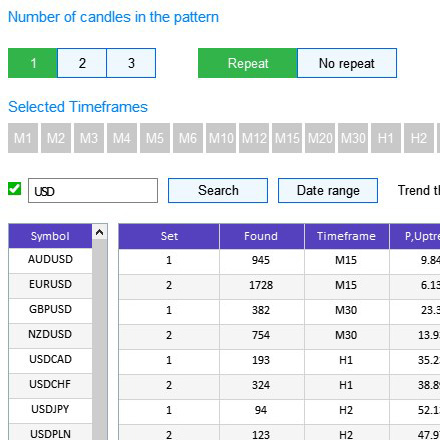Fig.9. Calculation example and order of result output in the table

As can be seen in Fig.9, the test was performed using the EURUSD currency pair, with the 1-candlestick pattern, on the M15, M30, H1 and H2 timeframes. Two simple candlesticks were selected for testing: with indexes 1 and 2. The implementation of the above described algorithm can be observed in the results table. It is performed as follows: first all generated patterns are analyzed one by one on the 15-minute timeframe, then on the 30-minute timeframe, and so on.

### Conclusion

The archive attached below contains all described files arranged into appropriate folders. For their proper operation, you only need to save the MQL5 folder into the terminal folder. To open the terminal root directory, in which the MQL5 folder is located, press the Ctrl+Shift+D key combination in the MetaTrader 5 terminal or use the context menu as shown in Fig 10 below.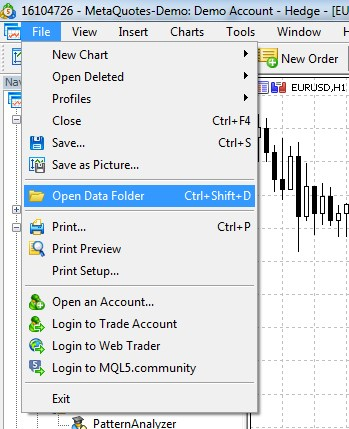Fig.10 Opening the MQL5 folder in the MetaTrader 5 terminal root.

Programs used in the article

#
Name
Type
Description
1
PatternAnalyzer.mq5 Graphical interface
Toolbar for analyzing candlestick patterns
2 MainWindow.mqh Code Base  GUI library
3 Program.mqh Code Base  Library of methods for creating UI and calculation elements

Previous articles in this series:

Studying candlestick analysis techniques (Part I): Checking existing patterns
Studying candlestick analysis techniques (Part II): Auto search for new patterns
Studying candlestick analysis techniques (Part III): Library for pattern operations

Translated from Russian by MetaQuotes Software Corp.
Original article: https://www.mql5.com/ru/articles/6301

Attached files |
MQL5.zip (495.94 KB)
Last comments | Go to discussion (5)| 27 Apr 2020 at 09:46

Dear Alexander, this is a beautiful pattern analysis tool! Thank you for sharing. I would love to give it a look but I am not able to start it.

I placed the archive content under MQL5 folder and compiled the PatternAnalyzer.mq5 with no errors.

Now, how do I start it? I tried to attach it to a EURUSD/D1 chart but it suddenly exits with error:  "Experts initializing of PatternAnalyzer (EURUSD,Daily) failed with code 1"

I've been able to start it in the Strategy Tester: the UI draws on the chart foreground but it doesn't seem to be usable (the buttons and combos are not clickable) as the mouse seems to act on the background chart.

I am using MT5 V5 build 2380. Any clue?

Thanks, Joe| 27 Apr 2020 at 10:21
Joe Baron:

Dear Alexander, this is a beautiful pattern analysis tool! Thank you for sharing. I would love to give it a look but I am not able to start it.

I placed the archive content under MQL5 folder and compiled the PatternAnalyzer.mq5 with no errors.

Now, how do I start it? I tried to attach it to a EURUSD/D1 chart but it suddenly exits with error:  "Experts initializing of PatternAnalyzer (EURUSD,Daily) failed with code 1"

I've been able to start it in the Strategy Tester: the UI draws on the chart foreground but it doesn't seem to be usable (the buttons and combos are not clickable) as the mouse seems to act on the background chart.

I am using MT5 V5 build 2380. Any clue?

Thanks, Joe

Hi, Check this version

<ex5 file deleted>| 27 Apr 2020 at 14:05
Alexander Fedosov:

Hi, Check this version

Dear Alexander, this one works like a charm!

Is there any chance to get the source code? I would really like to dive into it, understand the logic you've implemented and try to learn as much as possible on this practice (MT5 Programs development). I am a newbie.

In any case, let me thank you and wish you the best.

Joe| 27 Apr 2020 at 15:10
Joe Baron:

Dear Alexander, this one works like a charm!

Is there any chance to get the source code? I would really like to dive into it, understand the logic you've implemented and try to learn as much as possible on this practice (MT5 Programs development). I am a newbie.

In any case, let me thank you and wish you the best.

Joe

The article has all the sources. Study them.| 28 Apr 2020 at 08:20
Alexander Fedosov:

The article has all the sources. Study them.

OK sounds right.. I was just curious to understand why that one was failing to run.

I tried to debug it and found it exits with error while drawing one of the several buttons.. I wonder why!

In any case, thank you again!

Best

Joe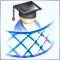Developing a cross-platform grider EA

In this article, we will learn how to create Expert Advisors (EAs) working both in MetaTrader 4 and MetaTrader 5. To do this, we are going to develop an EA constructing order grids. Griders are EAs that place several limit orders above the current price and the same number of limit orders below it simultaneously.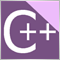A DLL for MQL5 in 10 Minutes (Part II): Creating with Visual Studio 2017

The original basic article has not lost its relevance and thus if you are interested in this topic, be sure to read the first article. However much time has passed since then, so the current Visual Studio 2017 features an updated interface. The MetaTrader 5 platform has also acquired new features. The article provides a description of dll project development stages, as well as DLL setup and interaction with MetaTrader 5 tools.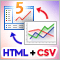How to visualize multicurrency trading history based on HTML and CSV reports

Since its introduction, MetaTrader 5 provides multicurrency testing options. This possibility is often used by traders. However the function is not universal. The article presents several programs for drawing graphical objects on charts based on HTML and CSV trading history reports. Multicurrency trading can be analyzed in parallel, in several sub-windows, as well as in one window using the dynamic switching command.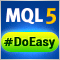Library for easy and quick development of MetaTrader programs (part IV): Trading events

In the previous articles, we started creating a large cross-platform library simplifying the development of programs for MetaTrader 5 and MetaTrader 4 platforms. We already have collections of historical orders and deals, market orders and positions, as well as the class for convenient selection and sorting of orders. In this part, we will continue the development of the base object and teach the Engine Library to track trading events on the account.